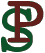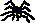The GLIBC random number generator
Written Jan 4, 2007.

The GNU C library's random() function provides pseudo-random numbers via a linear additive feedback method. A description of the exact algorithm used is hard to find, so I have documented it here.

The random(3) man page states, misleadingly, that "the random() function uses a non-linear additive feedback random number generator". This is not actually true, as the feedback is in fact linear modulo 232. The only slight non-linearity is introduced during the seeding stage, due to the fact that the seeding calculation is done modulo 231 - 1 and not modulo 231 or 232. This means that, although each generated random number depends linearly on previous numbers in the sequence, the random numbers as a whole do not depend linearly on the seed.

## Description of the algorithm

For reference, and because it is easier to read than source code, here is a mathematical description of the exact algorithm used by the GLIBC pseudo-random number generator. Note, in the following description, that 2147483647 = 231 - 1 and 4294967296 = 232. All quantities are mathematical integers.

For a given seed s, an initialization vector r0...r33 is calculated according to the following scheme:

• (1) r0 = s
• (2) ri = (16807 * (signed int) ri-1) mod 2147483647 (for i = 1...30)
• (3) ri = ri-31 (for i = 31...33)
Note that the multiplication by 16807 is done in a large enough signed integer type so that there is no overflow before the modulo operation. Also note that ri-1 is converted to a signed 32-bit value before the multiplication, but the only time this value can be negative is in the case of i=1, if s ≥ 231. The modulo operation is mathematical, i.e., the result is taken between 0 and 2147483646, even if ri-1 is negative.

Then a sequence of pseudo-random numbers r34... is generated by a linear feedback loop as follows:

• (4) ri = (ri-3 + ri-31) mod 4294967296 (for i ≥ 34)
r0...r343 are thrown away. The ith output oi of rand() is
• (5) oi = ri+344 >> 1
Note that this is a 31-bit number; the least significant bit of ri+344 is thrown away.

## Linearity

Despite the fact that the least significant bit is thrown away, we still have almost-linearity of the output sequence. As a consequence of equations (4) and (5), we have
• (6) oi = oi-31 + oi-3 mod 231 or oi = oi-31 + oi-3 + 1 mod 231, for all i ≥ 31.
Indeed, you can check this for yourself: the first 60 numbers returned by random() for seed 1 (the default seed), are:

 0: 1804289383 1: 846930886 2: 1681692777 3: 1714636915 4: 1957747793 5: 424238335 6: 719885386 7: 1649760492 8: 596516649 9: 1189641421 10: 1025202362 11: 1350490027
 12: 783368690 13: 1102520059 14: 2044897763 15: 1967513926 16: 1365180540 17: 1540383426 18: 304089172 19: 1303455736 20: 35005211 21: 521595368 22: 294702567 23: 1726956429
 24: 336465782 25: 861021530 26: 278722862 27: 233665123 28: 2145174067 29: 468703135 30: 1101513929 31: 1801979802 32: 1315634022 33: 635723058 34: 1369133069 35: 1125898167
 36: 1059961393 37: 2089018456 38: 628175011 39: 1656478042 40: 1131176229 41: 1653377373 42: 859484421 43: 1914544919 44: 608413784 45: 756898537 46: 1734575198 47: 1973594324
 48: 149798315 49: 2038664370 50: 1129566413 51: 184803526 52: 412776091 53: 1424268980 54: 1911759956 55: 749241873 56: 137806862 57: 42999170 58: 982906996 59: 135497281

And indeed,

• o31 = 1801979802 = o0 + o28 = 1804289383 + 2145174067 (mod 231)
• o32 = 1315634022 = o1 + o29 + 1= 846930886 + 468703135 + 1 (mod 231)
• etc.

## Simplified code

The following simple C program generates exactly the same sequence of pseudo-random numbers as the GLIBC random() function, for any given seed.
```#include <stdio.h>

#define MAX 1000
#define seed 1

main() {
int r[MAX];
int i;

r = seed;
for (i=1; i<31; i++) {
r[i] = (16807LL * r[i-1]) % 2147483647;
if (r[i] < 0) {
r[i] += 2147483647;
}
}
for (i=31; i<34; i++) {
r[i] = r[i-31];
}
for (i=34; i<344; i++) {
r[i] = r[i-31] + r[i-3];
}
for (i=344; i<MAX; i++) {
r[i] = r[i-31] + r[i-3];
printf("%d\n", ((unsigned int)r[i]) >> 1);
}
}
```
Note: the GLIBC implementation allows other degrees besides 31 in the linear feedback register, via the initstate() function. An analogous description applies in these situations.

Back to Homepage:Peter Selinger / Department of Mathematics and Statistics / Dalhousie University
selinger@mathstat.dal.ca / PGP key